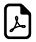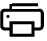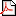Splitting Approach To Find Times Table FactsSplit Method Trick - All Times Table

An online common times table tricks.Easy way to learn all the times table.All Times Table Easy Learningcan write toResult
can write to
can write to
can write to
can write to
can write to
can write to
can write to
can write to
can write to
can write to

How split method works for math times table?

There are lot of ways to find the times table fact.The method described here is the splitting approach.Just look at our below split table generator and then try them yourself.The best way to learn is to have a go.

Example:

What is 12 x 3 = ?

Steps:

Split 12 into tens and units: 12 = 10 x 3 + 2 x 3 = 30 + 6 = 36....So, 12 x 3 = 36

Think Split.Get Times Table Fact Easily !!!

What math times time do you want to generate ?Times Table Challenge pdfbronze-times-table-challenge.pdf 29 Kb 27/02/17silver-times-table-challenge.pdf 29 Kb 27/02/17gold-times-table-challenge.pdf 29 Kb 27/02/17platinum-times-table-challenge.pdf 33s Kb 27/02/17Times Table Tricks 2 to 11►

2 Times Table Trick

4 Times Table Trick

5 Times Table Trick

6 & 7 Times Table Trick

8 Times Table TrickTop Calculators ►

Tax Calculator, Calories Burned Calculator, PERT, SD Calculator, Dog Age Calculator, Children Equal Playing Time Calculator